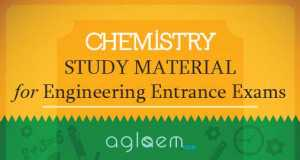## Chemistry Study Material for Engineering Entrance ExamsChemistry Study Material for Engineering Entrance Exams is given in 20 parts divided in chapters along with a revision article with important notes.

The topics from which chemistry questions are asked in engineering entrance exams are broadly divided into the chapters as given here. For students preparing for engineering entrance examinations, a firm understanding and rigorous practice of questions is essential to ace the exam. By studying the concept notes, explanation and examples of the chemistry topics of class 11 and 12, you can prepare well for your upcoming exams.

It is important to understand the theory, know about each important equation and laws and then have an in depth knowledge of problem solving. The chemistry study material given here helps accomplish all this in a comprehensive and accurate manner.

We urge you to first study the notes given in the introduction of each chapter and then try solving the chemistry problems given for practice. Once you have tried solving them, look up the answers, also given there. This way you can analyze your preparation and work your way ahead.